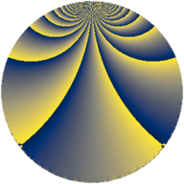# Properties

 Label 784.4.jLevel $784$ Weight $4$ Character orbit 784.j Rep. character $\chi_{784}(195,\cdot)$ Character field $\Q(\zeta_{4})$ Dimension $472$ Sturm bound $448$

# Related objects

## Defining parameters

 Level: $$N$$ $$=$$ $$784 = 2^{4} \cdot 7^{2}$$ Weight: $$k$$ $$=$$ $$4$$ Character orbit: $$[\chi]$$ $$=$$ 784.j (of order $$4$$ and degree $$2$$) Character conductor: $$\operatorname{cond}(\chi)$$ $$=$$ $$112$$ Character field: $$\Q(i)$$ Sturm bound: $$448$$

## Dimensions

The following table gives the dimensions of various subspaces of $$M_{4}(784, [\chi])$$.

Total New Old
Modular forms 688 488 200
Cusp forms 656 472 184
Eisenstein series 32 16 16

## Trace form

 $$472q + 4q^{2} - 16q^{4} - 92q^{8} + O(q^{10})$$ $$472q + 4q^{2} - 16q^{4} - 92q^{8} + 44q^{11} - 296q^{16} + 388q^{18} + 260q^{22} - 648q^{23} - 408q^{29} + 1132q^{30} + 984q^{32} + 176q^{36} - 12q^{37} + 8q^{39} - 816q^{43} - 188q^{44} - 760q^{46} - 768q^{50} - 1180q^{51} + 756q^{53} - 2860q^{58} + 1700q^{60} + 4472q^{64} + 8q^{65} - 188q^{67} + 432q^{71} + 4992q^{72} - 164q^{74} - 9212q^{78} - 33040q^{81} - 508q^{85} + 2112q^{86} + 4332q^{88} + 7580q^{92} - 212q^{93} - 4832q^{99} + O(q^{100})$$

## Decomposition of $$S_{4}^{\mathrm{new}}(784, [\chi])$$ into newform subspaces

The newforms in this space have not yet been added to the LMFDB.

## Decomposition of $$S_{4}^{\mathrm{old}}(784, [\chi])$$ into lower level spaces

$$S_{4}^{\mathrm{old}}(784, [\chi]) \cong$$ $$S_{4}^{\mathrm{new}}(112, [\chi])$$$$^{\oplus 2}$$